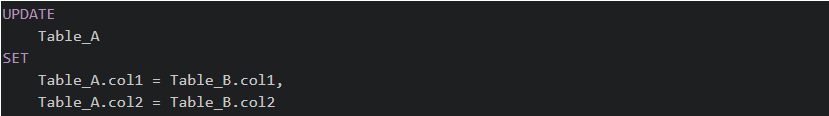# SQL - How do I UPDATE from a SELECT in SQL Server?INNER JOIN, MERGE, SQL, SQL Server, UPDATE

In SQL Server it's possible to INSERT into a table, but can you do the same for UPDATE? The is a common question when learning SQL and learning how to UPDATE from a SELECT in SQL Server.

In SQL Server 2008 (or better), use `MERGE`

``````MERGE INTO Table_A A
USING (
SELECT id, col1, col2
FROM Table_B
WHERE tsql = 'cool'
) B
ON A.id = B.id
WHEN MATCHED THEN
UPDATE
SET col1 = B.col1,
col2 = B.col2;``````

Otherwise you can use and `INNER JOIN`

``````UPDATE
Table_A
SET
Table_A.col1 = Table_B.col1,
Table_A.col2 = Table_B.col2
FROM
Some_Table AS Table_A
INNER JOIN Other_Table AS Table_B
ON Table_A.id = Table_B.id
WHERE
Table_A.col3 = 'cool'``````